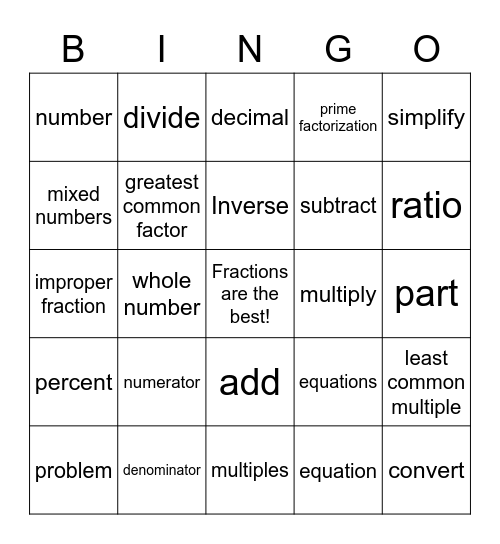# Fraction VocabularyThis bingo card has a free space and 24 words: numerator, denominator, Inverse, problem, simplify, number, mixed numbers, divide, multiply, add, subtract, improper fraction, equations, least common multiple, greatest common factor, equation, part, decimal, ratio, whole number, convert, percent, prime factorization and multiples.

## Play Online